# Reverse a linked list using Stack

## Introduction to the problem statement

We were given a linked list. The task is to use an auxiliary Stack to reverse the order of elements in the Linked List.

Example 1:

Input 1: 4 1 3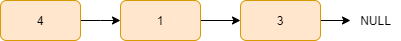Output 1: 3 1 4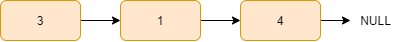Example 2:

Input 2: 4 7 8 1 9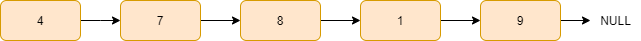Output 2: 9 1 8 7 4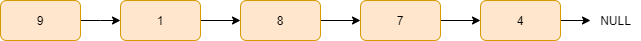## Approach

The idea of this approach is simple: traverse all the nodes one by one from the starting node of the given linked list and push all the values of the nodes into the auxiliary stack.

We know that the stack data structure follows the LIFO(last in first out) order. So, if we extract the content of the stack, we get the last element first, then the second last element and so on, i.e. we get the data in reverse order.

Finally, we change the linked list data from starting with the reversed data from the stack one by one for all nodes.

Steps:

1. Declare a stack.
2. Push all the values of the nodes from starting into the stack.
3. Change the values of the nodes from the beginning of the linked list with the top element of the stack.
4. Move to the next node and pop the top element from the stack, and
5. Repeat steps 3 and 4 while the stack is not empty.

Let’s understand the above approach with an example:

Initially, the curr pointer points to the head of the linked list, and all the nodes are pushed into the stack data structure.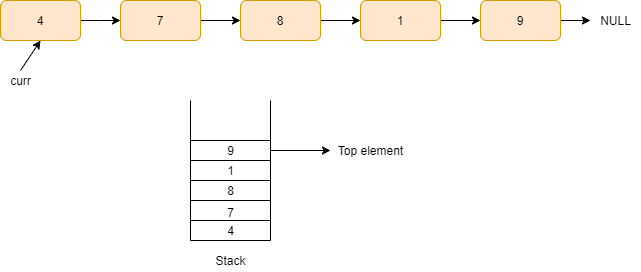Now we replace, curr->data with the stack.top() element and move the curr pointer to the next node and pop the top element of the stack.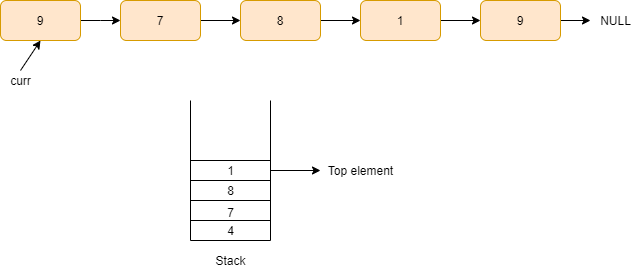Again repeat the above process by replacing curr->data with the stack.top() element and moving the curr pointer to the next node and popping the top element of the stack.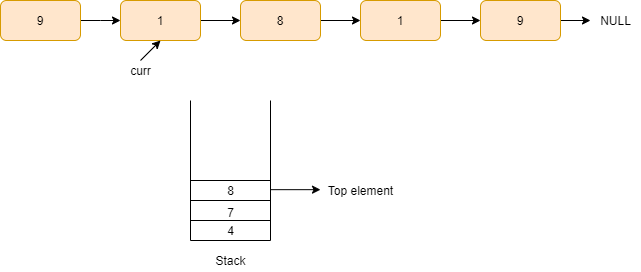We keep on repeating this step until we reach the end node of the linked list.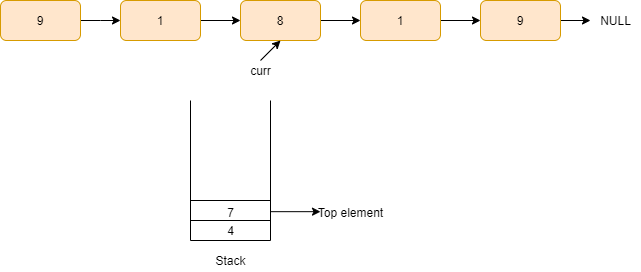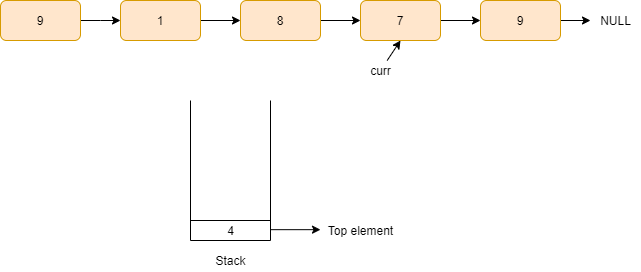Now, the stack is empty, and we get the required reversed linked list.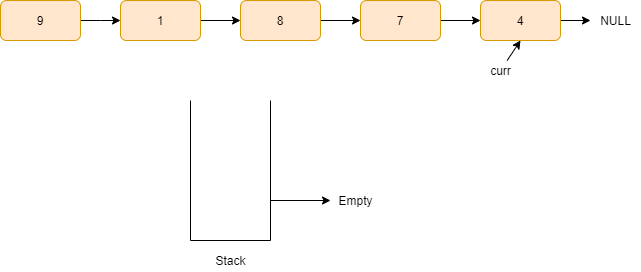## Code in C++

``````#include <bits/stdc++.h>
using namespace std;

struct Node {
int data;
Node* next;
Node(int x)
{
data = x;
next = NULL;
}
};

{
stack<int> st;

while (curr != NULL)
{
st.push(curr->data);
curr = curr->next;
}

while (!st.empty())
{
curr->data = st.top();
curr = curr->next;
st.pop();
}

}

void printList( Node* head, int nodes)
{

for (int i = 0; i < nodes; i++)
{
cout << curr->data << " ";
curr = curr->next;
}

}

int main()
{

int nodes = 5;

return 0;
}``````

Output

``9 1 8 7 4``

### Complexity Analysis

Time Complexity

O(n), where n is the number of nodes in the given linked list as we are traversing all the nodes at max.

Space complexity

It is O(n) as we are using a stack that contains all the nodes of the linked list.

1. Is stack LIFO or FIFO?
Stacks operate on the LIFO principle, which states that the element inserted last is the first element to be removed from the list. Whereas, Queues operate on the FIFO principle, which states that the element inserted first is the first element to be removed from the list.
2. What is the purpose of a linked list?
Linked lists are linear data structures that store information in individual nodes. These nodes contain information as well as a link to the next node in the list. Linked lists are commonly used due to their ease of insertion and deletion.

## Key Takeaways

So, this article discussed the approach to reverse a linked list with the help of stack data structure by using its LIFO order property, examples for a better understanding of the approach and its code in C++.

If you are a beginner, interested in coding and want to learn DSA, you can look for our guided path for DSA, which is free!

In case of any comments or suggestions, feel free to post them in the comments section. Happy Coding!